# Python Concurrency(iii)Photo by Michael Dziedzic on Unsplash

In the last blog we talked about Concurrency in Python, especially the Thread module. In this one, we are going to focus on the Multiprocessing module, and compare the differences with the Thread module.

## Multiprocessing — why ?

• It also has less need for synchronisation (like using Lock) ;
• Its child processes can be paused and terminated as it’s controlled by I/O;

But it has caveats like:

• Higher memory footprint;
• More expensive context switch

Compare the basic pattern of using Threading and Multiprocessing below, you will see little differences: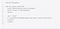Threading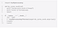Multiprocessing

Basically the main thread will initiate some worker/child threads to do the work and wait for it to finish.

Note the use of main dunder method though:

`if __name__ = “__main__”:`

This is for the sake of safe importing of main module, ie. make sure that the main module can be safely imported by a new Python interpreter without causing unintended side effects (such a starting a new process).

For example, using the spawn or forkserver start method running the following module would fail with a `RuntimeError`:

`from multiprocessing import Process def foo():   print(‘hello’) p = Process(target=foo) p.start()`

Instead one should protect the “entry point” of the program by using `if __name__ == '__main__':` as follows:

`from multiprocessing import Process, freeze_support, set_start_method def foo():    print(‘hello’) if __name__ == ‘__main__’:      freeze_support()      set_start_method(‘spawn’)      p = Process(target=foo)      p.start()`

This allows the newly spawned Python interpreter to safely import the module and then run the module’s `foo()` function.

A simple example of doing it is:

`from multiprocessing import Processdef func_a(name):    print('hello', name)def func_b(name):    print('hello', name)if __name__ == '__main__':    procs = []    p1 = Process(target=func_a, args=('bob',))    p1.start()    procs.append(p1)    p2 = Process(target=func_b, args=('jerry',))        p2.start()    procs.append(p2)    for p in procs:         p.join()`

## Pool

`from multiprocessing import Pooldef f(x):    return x*xif __name__ == '__main__':    with Pool(5) as p:        print(p.map(f, [1, 2, 3]))`

will print to standard output

`[1, 4, 9]`

Here `Pool.map()` applies the same function across the pool of child processes, and then waits until all function calls have completed before returning the list of results (If you are familiar with JavaScript, think of `Promise.all()` ).

Some of the key methods in Pool:

• `map（func, iterable[, chunksize])`a parallel equivalent of the `map()` built-in function. Note that both func and the iterable have to be pickable objects;
• `map_async` the async version of map;
• `apply` call func with arguments args. It blocks until the result is ready;
• `apply_async` It is better suited for performing work in parallel.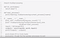map in a sync(blocking) operation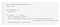apply in async operation

## Inter-process communication channels with Pipe and Queue

Pipe: Returns a pair (conn1, conn2) of Connection objects representing the ends of a pipe. Note that a pipe can be bidirectional or not depends on if duplex attribute is set to True or not.

Queue: Returns a process shared queue implemented using a pipe and a few locks/semaphores. When a process first puts an item on the queue a feeder thread is started which transfers objects from a buffer into the pipe. Queue implements all the methods of `queue.Queue` except for `task_done()` and `join()`.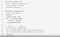inter-process using Pipe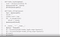inter-process using Queue

Also, it is important to note that there are multiple Queue methods in different modules. See below for comparison.

So when to use Pipe and when to use Queue?

• A `Pipe()` can only have two endpoints.
• A `Queue()` can have multiple producers and consumers.

If you need more than two points to communicate, use a `Queue()`. But if you need absolute performance, a `Pipe()` is much faster because `Queue()` is built on top of `Pipe()` (Not to mention the `JoinableQueue`)

## Synchronisation using Lock

`from multiprocessing import Process, Lockdef f(l, i):    l.acquire()    try:        print('hello world', i)    finally:        l.release()if __name__ == '__main__':    lock = Lock()    for num in range(10):        Process(target=f, args=(lock, num)).start()`

## Shared State using Manager and Value/List

There are two types of managing shared state: using shared memory or a Manager Process.

For shared memory, data can be stored in a shared memory map using `Value` or `Array`.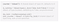Example shared memory

For example, the following code:

`from multiprocessing import Process, Value, Arraydef f(n, a):    n.value = 3.1415927    for i in range(len(a)):        a[i] = -a[i]if __name__ == '__main__':    num = Value('d', 0.0)    arr = Array('i', range(10))    p = Process(target=f, args=(num, arr))    p.start()    p.join()    print(num.value)    print(arr[:])`

will print

`3.1415927[0, -1, -2, -3, -4, -5, -6, -7, -8, -9]`

The `'d'` and `'i'` arguments used when creating `num` and `arr` are typecodes of the kind used by the `array` module: `'d'` indicates a double precision float and `'i'` indicates a signed integer. These shared objects will be process and thread-safe.

For more complicated shared resources, it is recommended to use a Manager Process.

A manager object returned by `Manager()` controls a server process which holds Python objects and allows other processes to manipulate them using proxies.

A manager returned by `Manager()` will support more types comparing to shared memory: `list`, `dict`, `Namespace`, `Lock`, `RLock`, `Semaphore`, `BoundedSemaphore`, `Condition`, `Event`, `Barrier`, `Queue`, `Value` and `Array`.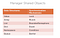List of data structures allowed in Manager

For example:

`from multiprocessing import Process, Managerdef f(d, l):    d = '1'    d['2'] = 2    d[0.25] = None    l.reverse()if __name__ == '__main__':    with Manager() as manager:        d = manager.dict()        l = manager.list(range(10))        p = Process(target=f, args=(d, l))        p.start()        p.join()        print(d)        print(l)`

will print

`{0.25: None, 1: ‘1’, ‘2’: 2} [9, 8, 7, 6, 5, 4, 3, 2, 1, 0]`

Server process managers are more flexible than using shared memory objects because they can be made to support arbitrary object types. Also, a single manager can be shared by processes on different computers over a network. They are, however, slower than using shared memory. As a result, if you only need simple data structures, use shared memory is preferable.

That’s so much of it today!

Happy Reading!